# Function Handle Assignment

This solution is perhaps a little bit fancier than it has to be, but illustrates what I want to show.

## Problem 1: The Lambert W function

I wrote a function called myLambert, which is at the bottom of this file. Here is how it works:
fprintf('I calculated W(2)=%0.4f.\n',myLambert(2));
I calculated W(2)=0.8526.
fprintf('MATLAB''s builtin lambertw function gives %0.4f.\n',lambertw(2))
MATLAB's builtin lambertw function gives 0.8526.

## Problem 2: Find all the zeros of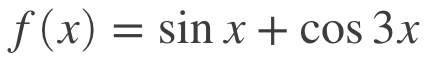First, let's just define the function and plot it to see what it looks like
f=@(x)(sin(x)+cos(3*x));
fplot(f,[0 2*pi])
xlabel('$x$');ylabel('$\sin{x}+\cos{3x}$')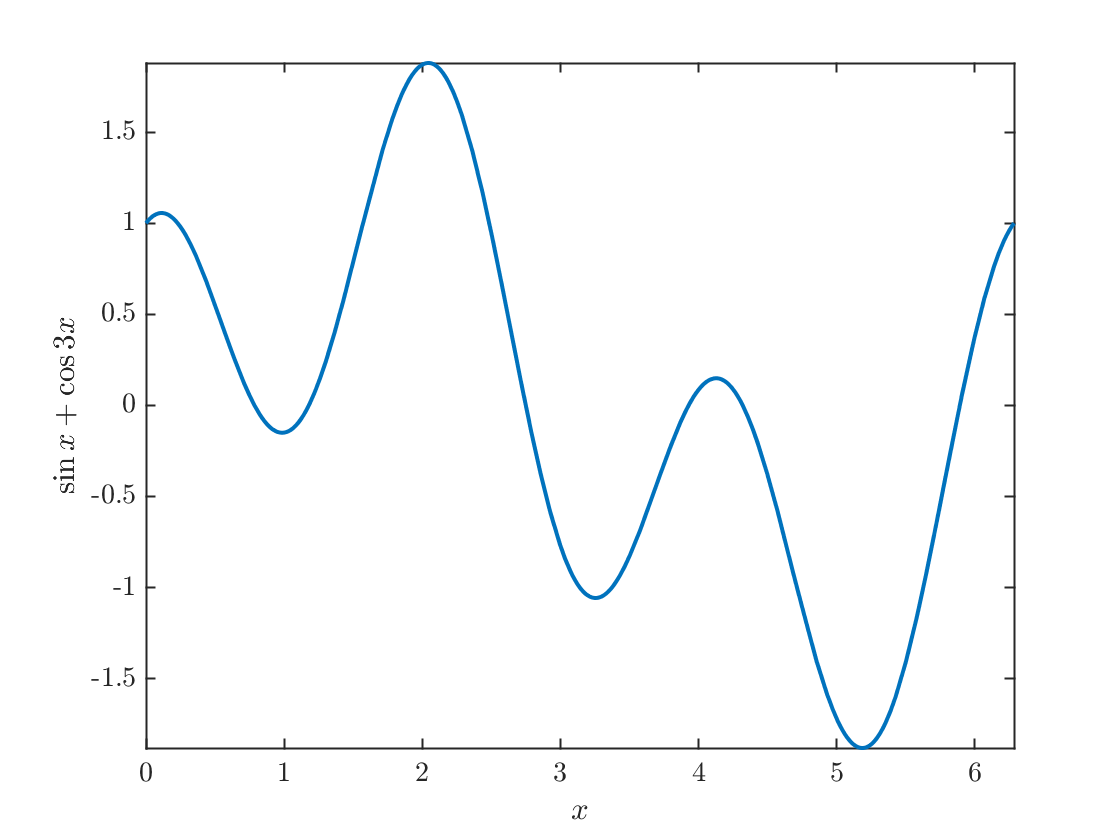I see six zeros. I will find them by defining an array of x values. If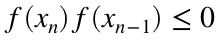, then there is a root on the interval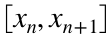.
x=linspace(0,2*pi,101);
ff=f(x);
spots=find(ff(1:end-1).*ff(2:end)<0);
Note that if the zero occurs at one of the endpoints, it will be double counted. Eliminate duplicates:
spots = unique(spots);
plot(x,ff,x(spots),ff(spots),'ro',x(spots+1),ff(spots+1),'rx');
title('The zeros are between the $\circ$ and $\times$ marks')
xlabel('$x$');ylabel('$\sin{x}+\cos{3x}$')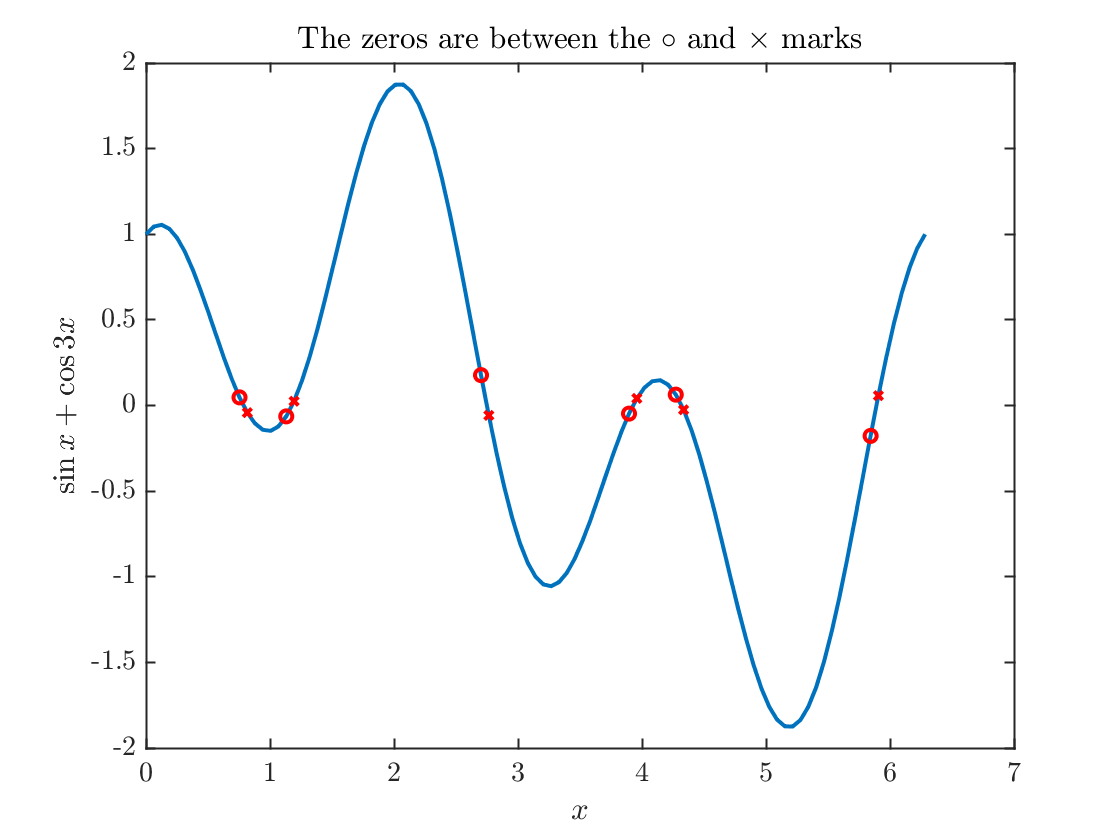xx=zeros(size(spots));
n=length(xx);
for k=1:n
xx(k)=fzero(f,x(spots(k):spots(k)+1));
end
plot(x,ff,xx,zeros(1,n),'o')
xlabel('$x$');ylabel('$\sin{x}+\cos{3x}$')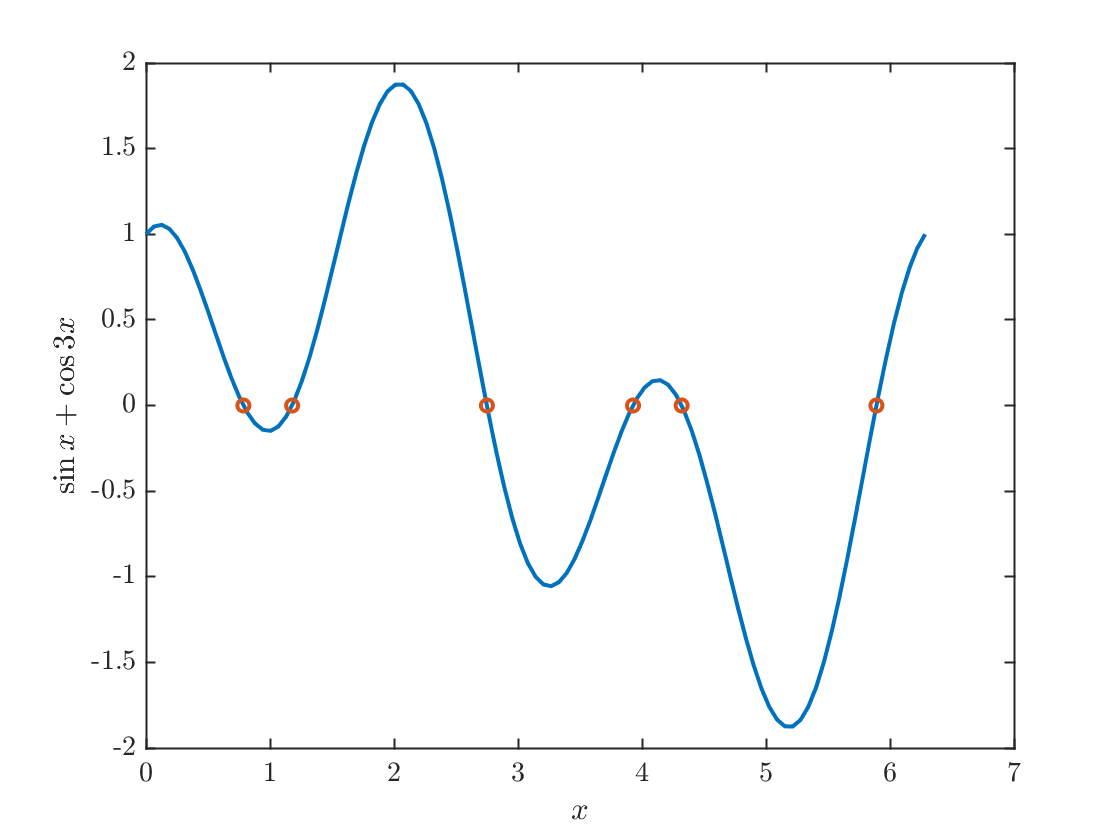fprintf('The zeros are:\n')
The zeros are:
fprintf('%6.3f\n',xx)
0.785 1.178 2.749 3.927 4.320 5.890

## The implementation of the Lambert-W function

function y=myLambert(xIn)
f=@(x)x.*exp(x)-xIn;
assert(xIn>-exp(-1),'xIn must be bigger than -1/e')
y=fzero(f,0);
end